## Hyperboloid

A Quadratic Surface which may be one- or two-sheeted.

The one-sheeted circular hyperboloid is a doubly Ruled Surface. When oriented along the z-Axis, the one-sheeted circular hyperboloid has Cartesian Coordinates equation(1)

and parametric equation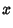(2)(3)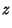(4)

for(left figure). Other parameterizations include(5)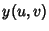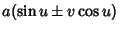(6)(7)

(middle figure), or(8)(9)(10)

(right figure). An obvious generalization gives the one-sheeted Elliptic Hyperboloid.

A two-sheeted circular hyperboloid oriented along the z-Axis has Cartesian Coordinates equation(11)

The parametric equations are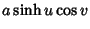(12)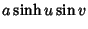(13)(14)

for. Note that the plus and minus signs in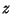correspond to the upper and lower sheets. The two-sheeted circular hyperboloid oriented along the x-Axis has Cartesian equation(15)

and parametric equations(16)(17)(18)

(Gray 1993, p. 313). Again, an obvious generalization gives the two-sheeted Elliptic Hyperboloid.

The Support Function of the hyperboloid of one sheet(19)

is(20)

and the Gaussian Curvature is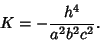(21)

The Support Function of the hyperboloid of two sheets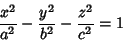(22)

is(23)

and the Gaussian Curvature is(24)

(Gray 1993, pp. 296-297).

Gray, A. The Hyperboloid of Revolution.'' §18.5 in Modern Differential Geometry of Curves and Surfaces. Boca Raton, FL: CRC Press, pp. 296-297, 311-314, and 369-370, 1993.